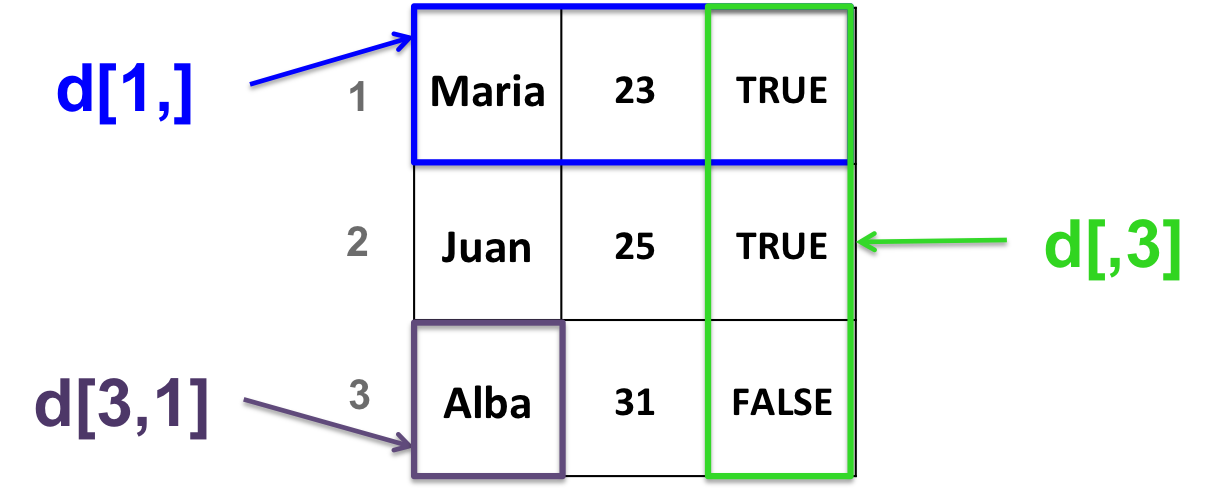## 9.6 Data frames

A data frame is a 2-dimensional structure.
It is more general than a matrix.

All columns in a data frame:

• can be of different types (numeric, character or logical)
• must have the same length

A data frame is organized by column: columns are variables and rows are observations of each variable.

### 9.6.1 Create a data frame

• With the data.frame function:
``````# stringsAsFactors: ensures that characters are treated as characters and not as factors
d <- data.frame(c("Maria", "Juan", "Alba"),
c(23, 25, 31),
c(TRUE, TRUE, FALSE),
stringsAsFactors = FALSE)``````
• Example why “stringsAsFactors = FALSE” is useful
``````# Create a data frame with default parameters
df <- data.frame(label=rep("test",5), column2=1:5)
# Replace one value
df[2,1] <- "yes"
# Throws an error and doesn't replace the value !``````
``````# Create a data frame with:
df2 <- data.frame(label=rep("test",5), column2=1:5, stringsAsFactors = FALSE)
# Replace one value
df2[2,1] <- "yes"
# Works!``````
• Converting a matrix into a data frame:
``````# create a matrix
b <- matrix(c(1, 0, 34, 44, 12, 4),
nrow=3,
ncol=2)
# convert as data frame
b_df <- as.data.frame(b)``````

### 9.6.2 Data frame manipulation:

Very similar to matrix manipulation.